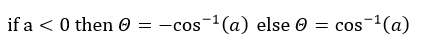# Solar Position

This collection of routines computes the solar position for points in time. The topocentric solar parameters are illustrated in Figure 4, which shows a panel tilted at angle β and offset from due southern orientation with an aspect angle γ.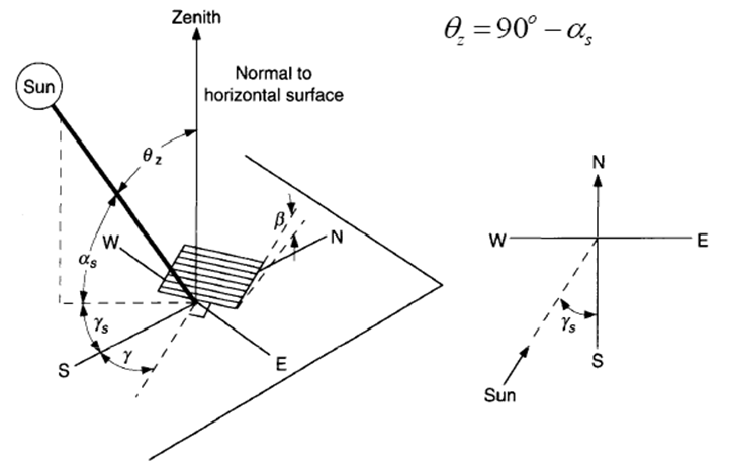### Figure 4. Solar Angles for a Tilted PV Panel

PlantPredict implements the Solar Position Algorithm (SPA) for Solar Radiation Applications by Reda and Andreas,
as described in the NREL report, TP-560-3402.

## Outputs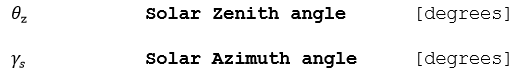## Algorithm

The SPA is implemented as-is, with ΔT: TT-UT (difference between the Earth rotation time and the Terrestrial
Time), assumed to be fixed at 67s.

## References

Reda, I., Andreas, A. Solar Position Algorithm for the Solar Radiation Applications. NREL Technical Report NREL/TP-560-3402

# Site Pressure

This function empirically computes the nominal station pressure given the site elevation.

## Inputs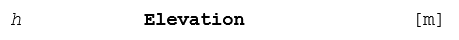## Outputs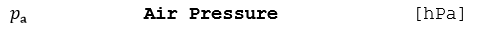## Algorithm 1 (Physical)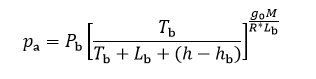## Algorithm 2 (Empirical)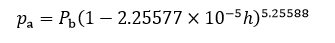## Where: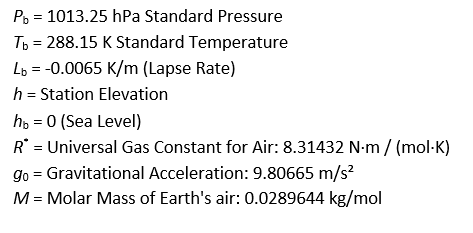## References

Wallace, John M., and Peter Victor Hobbs. Atmospheric Science: An Introductory Survey. Pp. 55-60 1977, pp 55-60

# Sunrise & Sunset

This routine computes the approximate sunrise and sunset times given the site coordinates and the declination angle, previously computed as part of the solar position equation.

## Inputs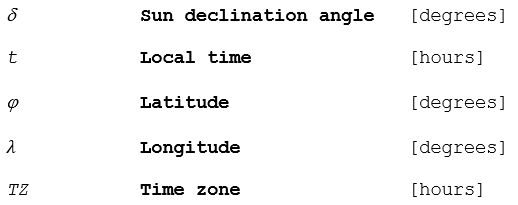## Outputs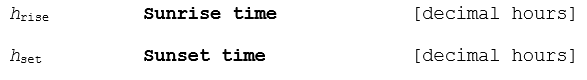## Algorithm

1.) Find the sunrise and sunset times assuming the standard refraction of the sun at the horizon with the midpoint
of the solar disk about 0.833° below the horizon.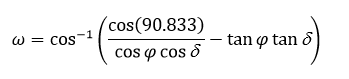2.) Find the sunrise and sunset solar times.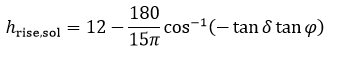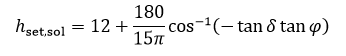3.) Convert from solar to local time by subtracting the equation of time difference instead of adding it.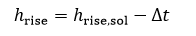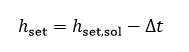## References

http://www.srrb.noaa.gov/highlights/sunrise/solareqns.PDF

# Atmospheric Refraction Correction

This function computes the apparent shift in the solar elevation angle caused by atmospheric refraction. Note that the output is a positive number; i.e. near the horizon, the solar disk appears higher in the sky than its true position. The apparent elevation is the true (geometrical) elevation plus the correction factor.

## Inputs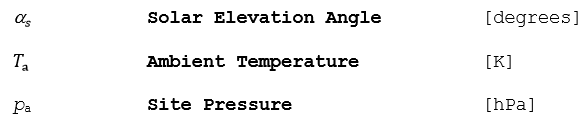## Outputs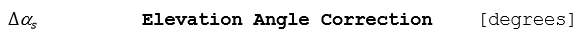## Algorithm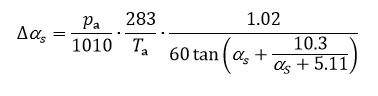### Where: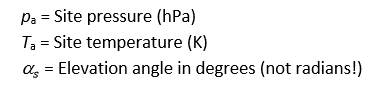## References

Reda, I., Andreas, A. Solar Position Algorithm for the Solar Radiation Applications. NREL Technical Report NREL/TP-560-3402

# Tilt Angle

Three different types of DC Field tilt technologies are supported by PlantPredict: fixed tilt, seasonal tilt, and horizontal-axis tracking. These three are mutually exclusive and can be configured as part of the DC Field definitions. They impact the available plane-of-array irradiance and row-on-row shading.

# DC Field Fixed Tilt

This is the base case. The tilt angle of the DC Field is static and set for all simulation time.

### Inputs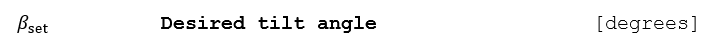### Outputs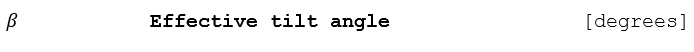### Algorithm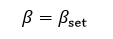# Seasonal Tilt

This is a variation of the fixed-tilt case, where the effective tilt angle depends on the 12-month lookup table of desired tilt angles as defined in the DC Field definitions.

### Inputs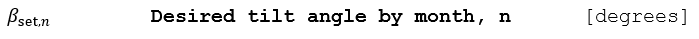### Outputs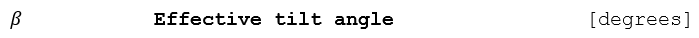### Algorithm

Set the instantaneous tilt angle for time t according to the by-month lookup of array tilt angles, where n=1:12: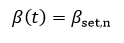# Tracking

For horizontal north-south axis tracker, this procedure computes the ideal tracker angle φτ, taking into consideration backtracking shade avoidance strategy and the tracker’s mechanical limits of travel. This procedure is generalized for a tracker whose N-S axis is skewed by a tracker yaw angle γτ (azimuth angle).

The coordinate system used in this suite of algorithms is the “North-East-Down” convention (NED), as follows:

• Solar azimuth angle is measured from North (N=0°, E=90°, S=180°, W=270°)

The other angles are positive when rotating counter-clockwise looking towards the origin.

• Tracker roll (tilt) angle is positive East, negative West, 0° is horizontal (facing up)
• Tracker yaw angles are positive east (e.g. 0° is N-S; 10° has a slight NNW-SSE orientation)

This is illustrated in Figure 5.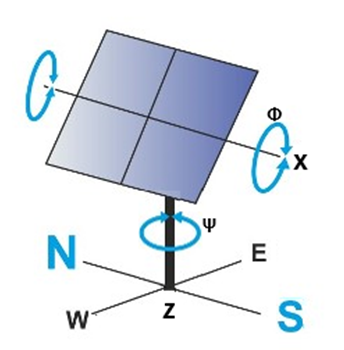### Figure 5. Modified Tracker Coordinate System

This procedure returns the tracker angle, as well as the effective tilt and azimuth angles, rotated in the reference
frame of a basic fixed-tilt array. In the case of a horizontal north-south tracker with a yaw angle of 10° degrees
east with the tracker set at an evening angle 45° to the east, the effective tilt β and azimuth γ would
be +45° be and +80°, respectively. The derivation of the rotation angle for optimum tracking of a single-axis

PlantPredict only supports a horizontal tracker (where the pitch angle is zero). This section summarizes the referenced equations into a form easily implanted in source code.

Note: Unlike in the NREL technical report, the incidence angle is calculated separately in order to have a generalized solution for both fixed-tilt and tracking array configurations.

## Inputs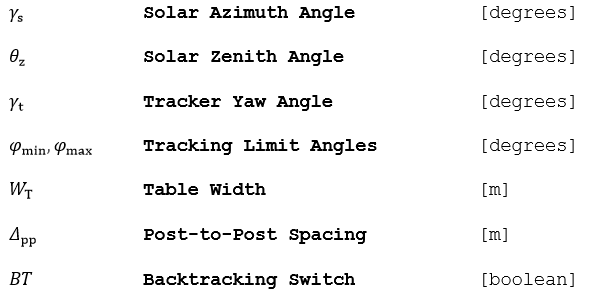## Outputs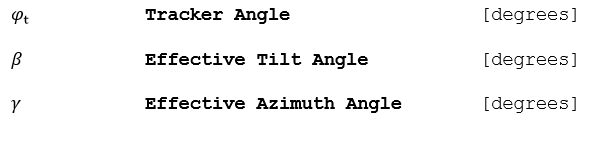## Algorithm (True Tracking)

1.) Convert the solar azimuth and yaw angles into the NED reference frame.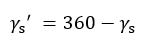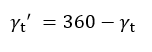2.) Find the ideal tracker angle, with no backtracking or mechanical stops.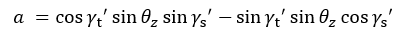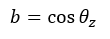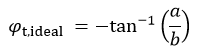3.) If the solar azimuth angle is not between (-90°, +90°), switch the sign of the ideal tracker angle: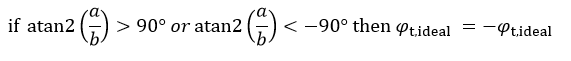## Algorithm (Backracking)

If backtracking is activated (as it would typically be for PV technologies using bypass diodes), then compute
the modified tracker angle with shade avoidance:

1.) Find the cutoff tracking angle where the row-to-row shading begins, which depends on the ground coverage
ratio (tracker width / post-to-post spacing)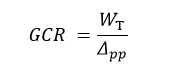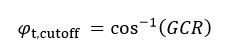2.) If the cutoff angle is not reached, then tracking is unaffected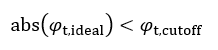3.) Else, invoke the backtracking algorithm, taking care of the sign of the tracker angle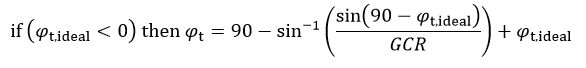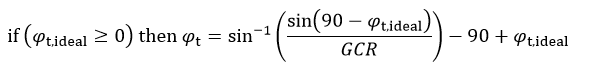## Algorithm (Tracker Limits)

1.) If the tracker limits are reached, the tracker stops moving.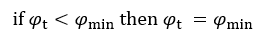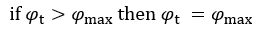2.) Report the effective tilt and azimuth angles. An east-west tracker is defined to have an azimuth of 0°
if the tracker axis is north-south (azimuth is defined relative to the tracking axis, not the collector);
hence in the morning, the tracker has an effective collector azimuth of 90° (facing east), and in the afternoon,
the effective collector azimuth is 270° (facing west).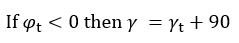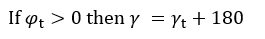3.) Report the effective tilt angle as the absolute value of the tracker angle (simplification only valid for
horizontal trackers).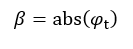## Reference

Marion, William F. and Dobos, Aron P. “Rotation Angle for the Optimum Tracking of One-Axis Trackers.”NREL. July 2013. NREL/TP-6A20-58891, 2003

# Compute Incidence Angle

This function computes the solar angle of incidence on the plane-of-array, i.e. the angle between a line perpendicular (normal) to the module surface and the beam component of the sunlight. The tilt may be constant for fixed-tilt DC Fields, or variable for tracking DC Fields.

## Inputs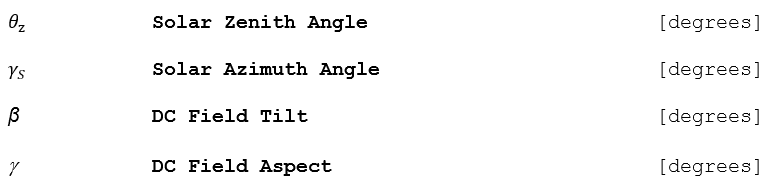## Outputs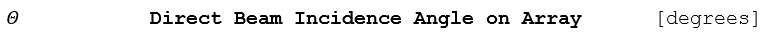## Algorithm

1.) Compute the incidence angle given an array of panel tilts β and aspects γ. The angle is defined
to be zero when the light ray is normal to the surface.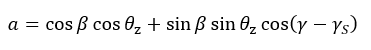2.) Preserve the sign of the incidence angle to determine if the beam is behind the plane of the array.